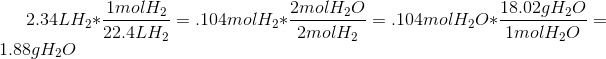# College Chemistry : Stoichiometric Calculations and Dimensional Analysis

## Example Questions

### Example Question #1 : Stoichiometric Calculations And Dimensional Analysis

Consider the unbalanced equation for the combustion of pentane: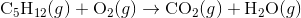How many grams of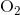is required to react with exactly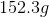of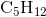.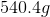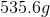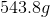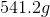Explanation:

Start by balancing the given chemical equation: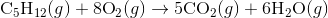Next, convert the grams of pentane into moles of pentane.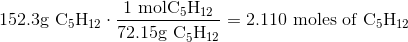Use the stoichiometric ratio given by the balanced equation to find the number of moles of oxygen needed to react with the pentane.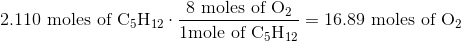Finally, convert the number of moles of oxygen into grams of oxygen.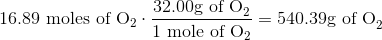There should besignificant figures, so the answer is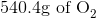.

### Example Question #1 : Stoichiometric Calculations And Dimensional Analysis

Consider the following unbalanced reaction: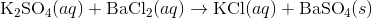How many grams of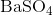can be produced if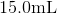of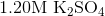is mixed with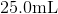of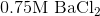?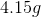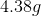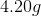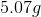Explanation:

Start by balancing the equation: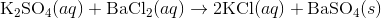Next, figure out which reactant is the limiting reactant.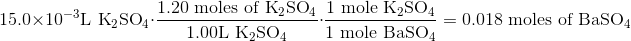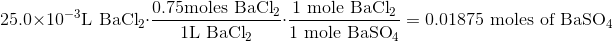Since fewer moles ofis produced when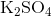is reacted, thenmust be the limiting reactant.

Convert the amount of moles ofinto grams.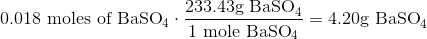### Example Question #1 : Stoichiometric Calculations And Dimensional Analysis

What is the mass in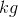of a gas with a volume of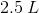and a density of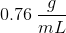?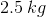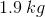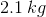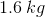Explanation:

For this question, we're given the density and volume of a gas and we're asked to find the mass of the gas.

To answer this question, we'll need to use dimensional analysis. What this means is that we'll need to cancel out units in order to obtain the ones that we're looking for.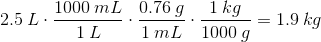### Example Question #1 : Stoichiometric Calculations And Dimensional AnalysisUse the equation shown to determine how many grams of water form when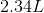of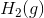reacts completely with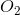at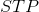.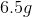of water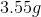of water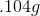of water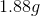of waterof water
Use the relations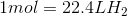, molar mass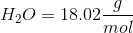, and